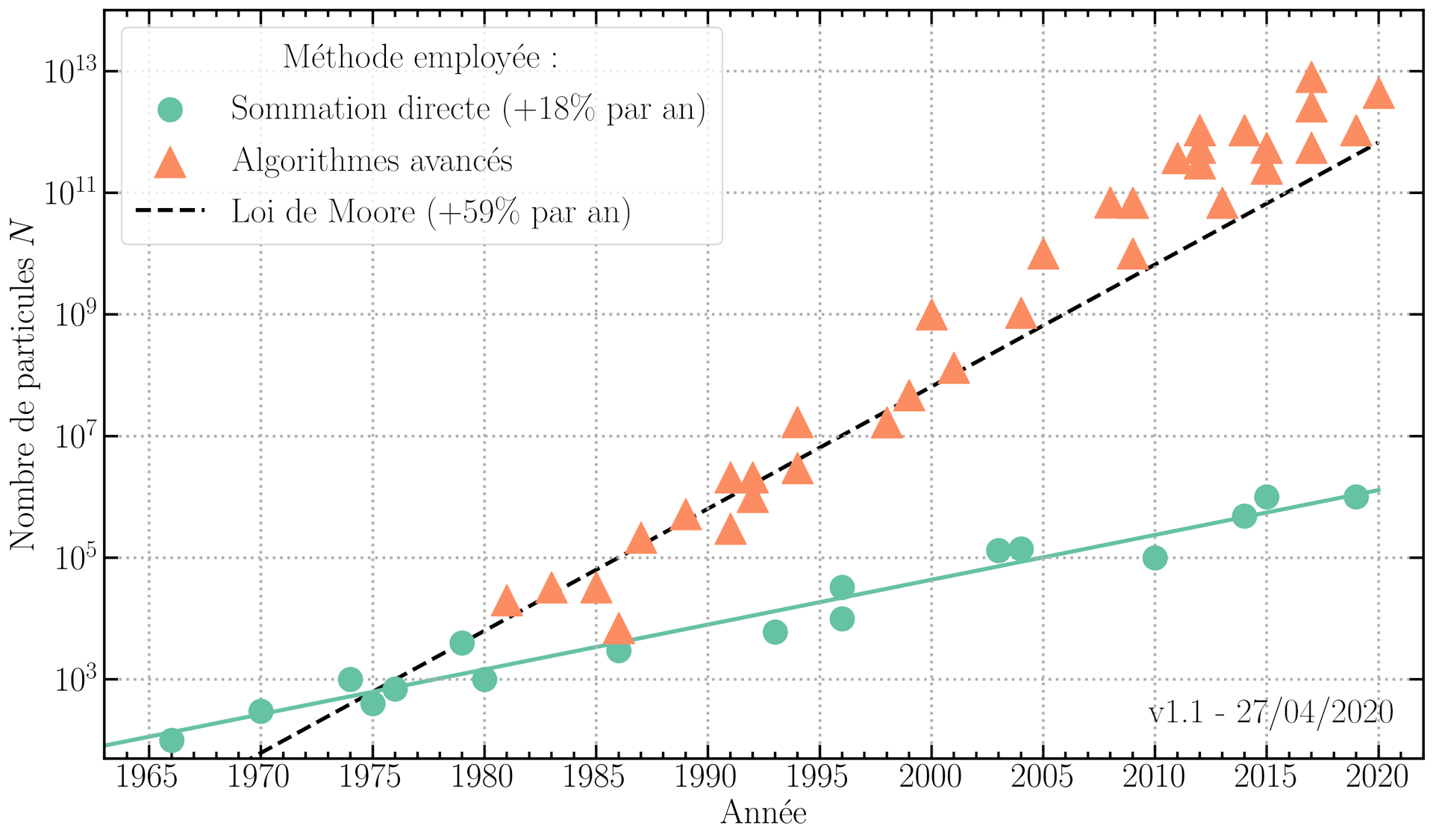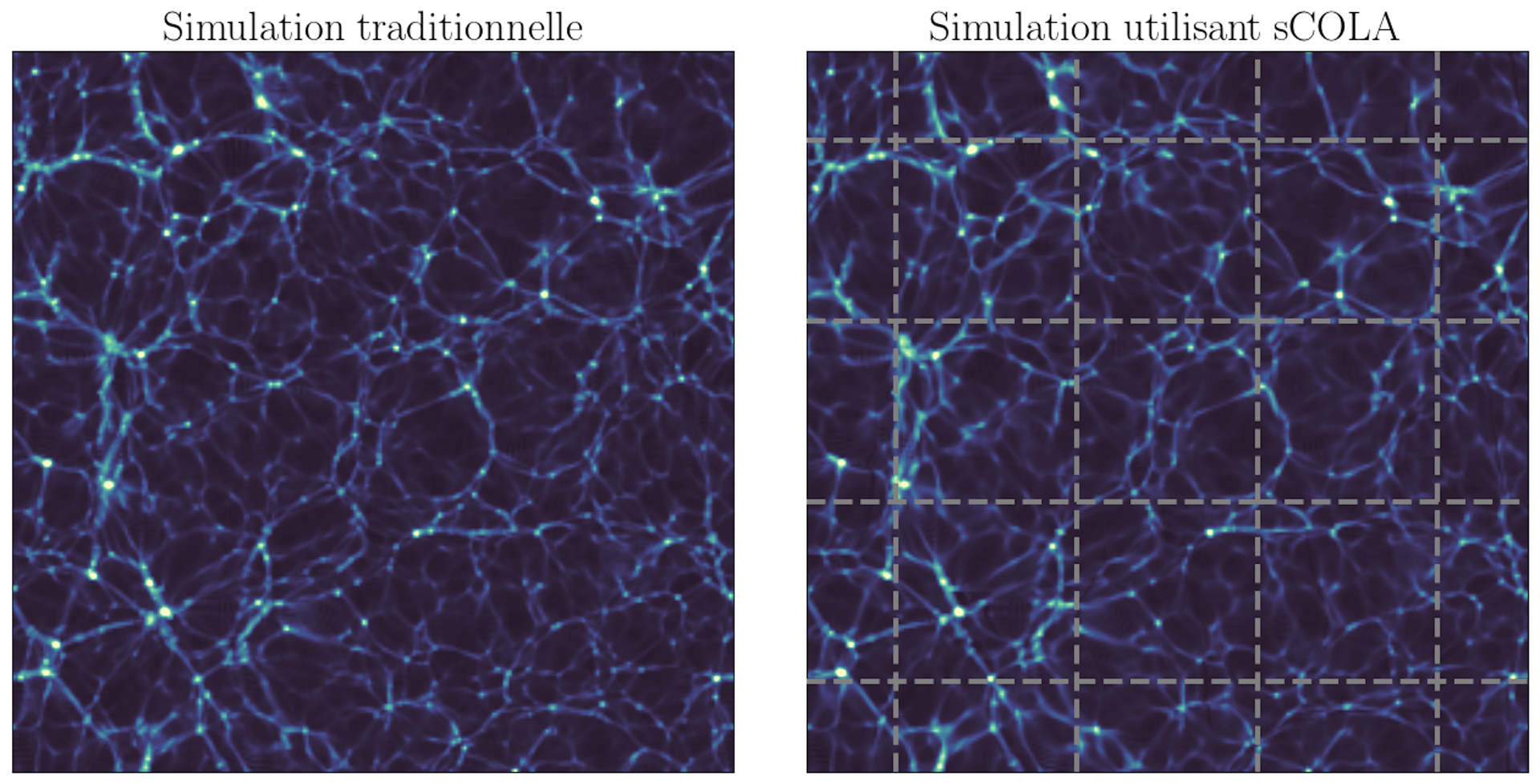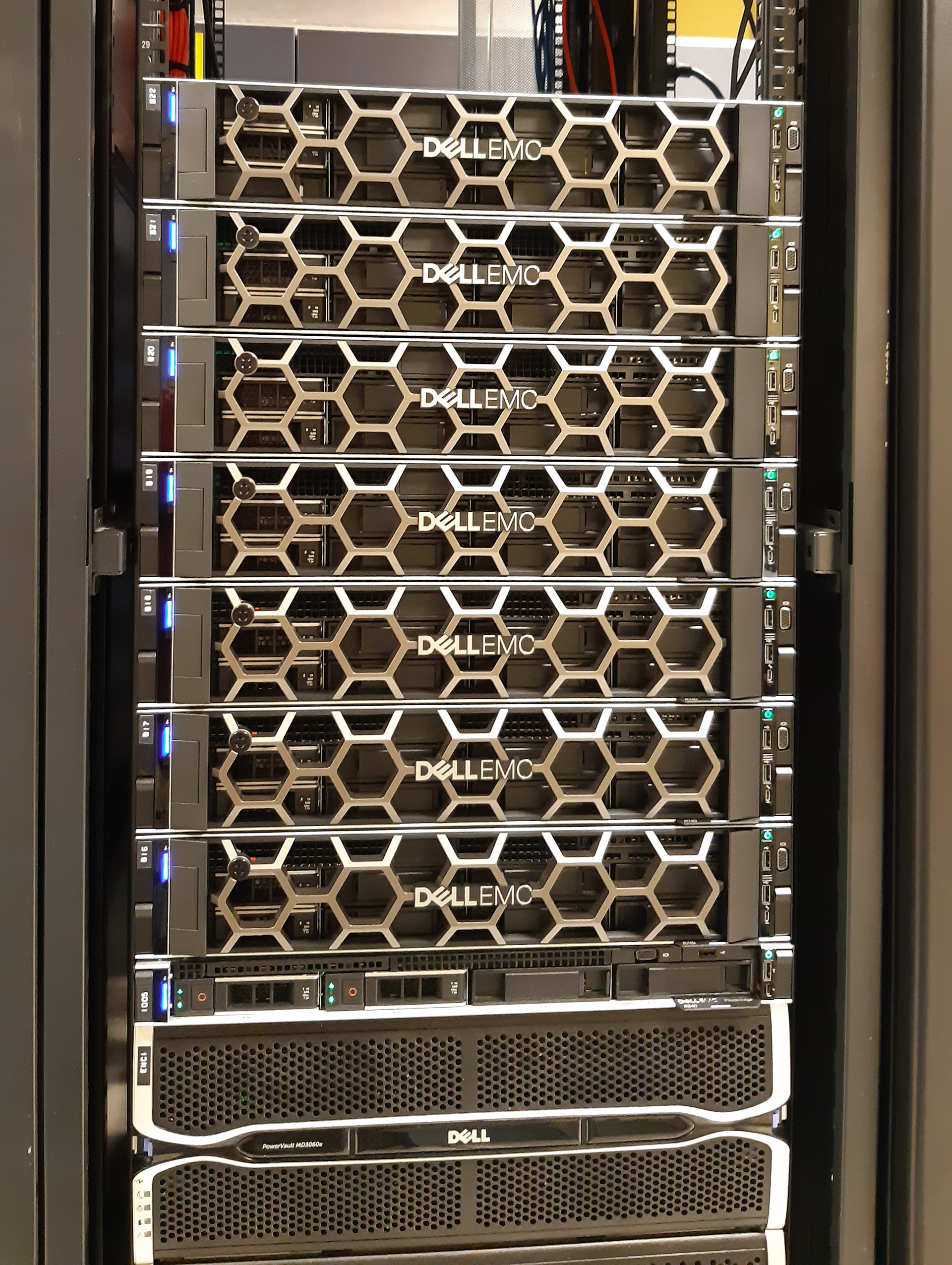# Towards a simulation of the Universe on a mobile phone

There are about two trillion galaxies observable in the Universe, and the evolution of each is sensitive to the presence of all the others. Faced with such gigantism, how to understand and calculate the evolution of the Universe?

Oith stars and galaxies, these objects that we can see, the Universe is filled with objects invisible to the naked eye, for example pulsar which emit in invisible frequency domains. There are also objects that by definition do not emit or absorb light, and therefore difficult to observe - these are dark matter and dark energy. These visible and invisible objects create clusters in space that evolve over time, forming a gigantic filamentary structure called the "Cosmic web".

The physical challenges

Connecting our knowledge of physics - especially the equations that govern the evolution of dark matter and dark energy - to data on the positions and light spectra of galaxies requires considerable computational resources. The most recent observations cover absolutely gigantic volumes: of the order of that of a cube 12 billion light years apart. Since the typical distance between two galaxies is only a few million light years, this leads us to simulate about a trillion galaxies to reproduce the observations. In the next ten years, the mission Euclid and the observatory Vera Rubin will provide information on several billion galaxies.

To be able to follow the physics of the formation of these galaxies, the spatial resolution would have to be of the order of ten light years. Ideally, then, the simulations should have a "scale ratio", close to a billion - that is, the largest physical scale of the problem is a billion times greater than the smallest physical scale of the problem. problem. No computer in existence or even under construction can achieve such a goal.

In practice, it is therefore necessary to have recourse to approximate techniques, consisting in "populating" the simulations with fictitious but realistic galaxies. This approximation is all the more justified since the calculation of the evolution of the components of a galaxy, for example the stars and interstellar gas, brings into play phenomena that are very fast compared to the global evolution of the cosmos. The use of fictitious galaxies nevertheless requires simulating the dynamics of the universe with a scale ratio of the order of 4, which current supercomputers just allow to do.

Algorithmic and material developments have drastically improved cosmological simulations

Simulating the gravitational dynamics of the Universe is what physicists call a body problem. Although the equations to be solved are all analytical as in most cases in physics, the solutions do not have a simple expression and require numerical techniques as soon as 𝑁 is greater than four. The direct numerical solution consists in explicitly calculating the interactions between all the pairs of “particles”, also called “bodies” (hence the name of problem with 𝑁 bodies).

To solve this problem, the computation of the forces "by direct summation" was privileged in cosmology at the beginning of the development of numerical simulations, in the years 1970. With this method, the number of necessary operations increases as 𝑁2, the square of the number of bodies, which counterbalances the material progress, like the use of graphics cards for the parallel computation.Figure 1: Evolution of the number of particles used in the body simulations according to the year of publication. The different symbols and colors correspond to different methods used to solve gravitational dynamics (direct summation in green, advanced algorithms in orange). For comparison, Moore's law concerning the power of computers is represented by the black dotted line.

In order to reduce the digital cost of simulations, most of the work in digital cosmology since 1980 has consisted in improving algorithms. The goal was to do away with the explicit calculation of all the gravitational interactions between particles, in particular for the pairs which are the furthest apart in the volume to be simulated. These algorithmic developments have allowed a gigantic increase in the number of particles used in cosmological simulations, in orange in figure 1. And in fact, since 1990, the increase in computing capacities in cosmology has been. faster that Moore's law, the software improvements adding to the increase in the performance of the computers!

The current limit: the slowness of communications between processors

In 2020, with the architectures of current supercomputers, the calculations are no longer limited as before by the number of operations that the processors can perform in a given time, but by the intrinsic slowness of the communications between the different processors involved in the so-called "parallel" calculations.

In these calculation techniques, a large number of processors work together and synchronously to perform a calculation that is much too complex to be performed on a conventional computer. The performance cap due to latencies in communications between processors was theorized as early as 1967 in the "Amdahl's law", from the name of the computer scientist who formulated it. Improving the "parallelism" of algorithms is now the main challenge for cosmological simulations.

The sCOLA approach: better divide and conquer

Let us return to the physical problem to be solved: it is a question of simulating the gravitational dynamics of the Universe at different scales. On a “small” scale, there are many objects that interact with each other: digital simulations are essential. At "large" spatial scales, that is to say if we look at Figure 2 from a great distance, not much happens during evolution (except a linear increase in amplitude inhomogeneities). Despite this, with a traditional simulation algorithm, the gravitational effect of all the particles on each other must be calculated, even if they are very far apart. It is expensive and almost useless, because most of the gravitational evolution is correctly described by simple equations, the resolution of which can be done without a computer.Figure 2: Comparison between a traditional simulation (left) and a simulation using our new algorithm (right). In our approach, the volume of the simulation is a mosaic made up of independently calculated “squares” whose edges are represented by dotted lines. F.Leclercq

In order to minimize unnecessary numerical calculations, we use a hybrid simulation algorithm (sCOLA): analytical at large scales and numerical at small scales, where interactions body interactions are important. The underlying idea is usual in physics, it is a "Change of reference" : the large-scale dynamics are taken into account by the new frame of reference, while the small-scale dynamics are entrusted to the computer which solves it by classical calculations of the gravity field.A computer based on graphics cards (GPUs) such as can be found at the Institut d'Astrophysique de Paris. It is only one hundredth of the cost of a national supercomputer. G.Lavaux

Moreover, this concept makes it possible to “divide and conquer better”, by simulating small sub-volumes independently, without communication with neighboring sub-volumes. Our approach therefore makes it possible to represent the Universe as a large mosaic: each of the “tiles” is a small simulation that a modest computer can solve, and the assembly of all the tiles gives the whole picture. This is the first time that cosmological simulations are perfectly parallel, and we have thus obtained simulations of a size comparable to that of the observable Universe, at a satisfactory resolution, while remaining on a laboratory computing center.

New calculators to simulate the Universe

This new algorithm makes it possible to envision new ways of exploiting computers: each of the "tiles" could be small enough to fit in the "Cache memory" of our calculators, the part of memory that processors can access the fastest, which would increase the speed of calculation and simulate the entire volume of the Universe extremely quickly, or at a resolution never before achieved.

We can finally imagine that each of the simulations corresponding to a "tile" is small enough to be executed on a mobile phone, making it possible to perform distributed computing collaborative as with the platform Cosmology @ Home.

The Ile-de-France Region finances research projects in areas of major interest and is committed through the Paris Region Phd system to the development of doctorates and training through research by co-financing 100 doctoral contracts from here 2022. For more information, visit iledefrance.fr/education-recherche.Florent Leclercq, Imperial College Research Fellow, Imperial College London et Guilhem-Lavaux, Researcher at IAP, labeled Area of ​​Major Interest by the Île-de-France Region, Paris Institute of Astrophysics